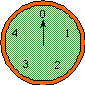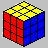# Introduction to Abstract Algebraby Henri Picciotto

I have taught these lessons in one form or another to students in Kindergarten through 12th grade, and to teachers, since 1971. Most recently, they have played a crucial role in Space, an advanced high school geometry elective I taught at the Urban School of San Francisco.

Taken together, these lessons are a good introduction to the power and beauty of mathematical structure. The approach is playful and founded on student experience and reflection. The key concept is that of a group, with a special emphasis on the identity and inverse elements, which are essential understandings throughout K-12 mathematics.

Download the following PDF files, which contain the version of these lessons that was published in 1994 in Algebra: Themes, Tools, Concepts (ATTC), the textbook I co-authored with Anita Wah.
Lessons
Teacher Notes
Helpful handouts: ducats.pdf | triangle.pdf
The much increased emphasis on geometric transformations in the Common Core State Standards should result in additional relevance for these lessons at the pre-college level.

The following worksheets for math teachers take the ideas of the above lessons one step further by introducing the concept of isomorphism and the definition of a field. This leads to the construction of the integers and rational numbers. These activities introduce teachers to a generalization and formalization of the arithmetic and algebra they teach. Moreover, they offer a glimpse at the part of university mathematics that is most relevant to teachers' practice.